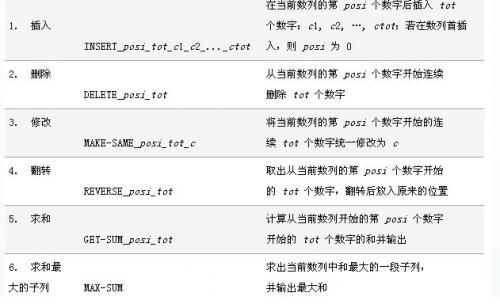# 题目大意

• $I$ $x$ $a$ : 在第$x$个字符后面插入字符$a$

• $D$ $x$ $y$：删除从$x$到$y$的这一段

• $C$ $x$ $y$ $z$ ：复制从$x$到$y$这一段，粘贴到第$z$个字符后面

• $P$ $x$ $y$ $z$ ：打印$x$次修改操作之前，从$y$到$z$这一段。其中，$x$不超过一个给定的数$M$。

# 题目大意